Queues-and-Stacks

 Question 1
The five items: A, B, C, D, and E are pushed in a stack, one after other starting from A. The stack is popped four items and each element is inserted in a queue. The two elements are deleted from the queue and pushed back on the stack. Now one item is popped from the stack. The popped item is
 A A B B C C D D
Data-Structures       Queues-and-Stacks       ISRO-2007
Question 1 Explanation:
When five items: A, B, C, D, and E are pushed in a stack: Order of stack becomes: A, B, C, D, and E (A at the bottom and E at the top.) stack is popped four items and each element is inserted in a queue: Order of queue: B, C, D, E (B at rear and E at the front) Order of stack after pop operations = A. Two elements deleted from the queue and pushed back stack: New order of stack = A, E, D(A at the bottom, D at the top) As D is on the top so when pop operation occurs D will be popped out. So, correct option is (D).
 Question 2
The best data structure to check whether an arithmetic expression has balanced parenthesis is a
 A Queue B Stack C Tree D List
Data-Structures       Queues-and-Stacks       ISRO-2017 May
Question 2 Explanation:
→ The stack is the best data structure to validate the arithmetic expression.
→ While evaluating when left parentheses occur then it pushes into the stack when right parentheses occur pop from the stack. While at the end there is empty in the stack.
 Question 3
The following postfix expression with single digit operands is evaluated using a stack:
8 2 3 ^ / 2 3 * + 5 1 * –
Note that ^ is the exponentiation operator. The top two elements of the stack after the first * is evaluated are
 A 6,1 B 5,7 C 3,2 D 1,5
Data-Structures       Queues-and-Stacks       ISRO-2016
Question 3 Explanation:
Expression: 8 2 3 ^ / 2 3 * + 5 1 * –
Rule (1): If the element is a number, Push it into stack.
Rule (2): If the element is an operator, Pop operands from the stack. Evaluate the operator operation and Push the result back into the stack.Question 4
The five items: A, B, C, D, and E are pushed in a stack, one after other starting from A. The stack is popped four items and each element is inserted in a queue. The two elements are deleted from the queue and pushed back on the stack. Now one item is popped from the stack. The popped item is
 A A B B C C D D
Data-Structures       Queues-and-Stacks       ISRO CS 2009
Question 4 Explanation: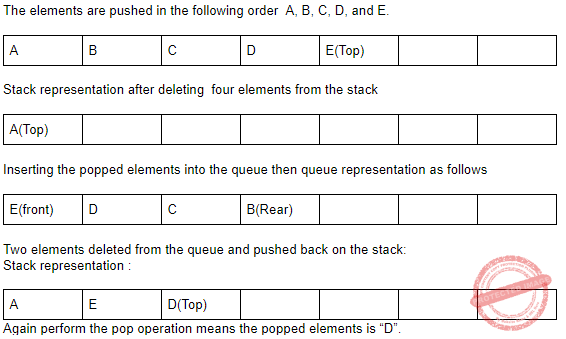Question 5
Consider a standard Circular Queue ‘q’ implementation (which has the same condition for Queue Full and Queue Empty) whose size is 11 and the elements of the queue are q, q, q…..,q. The front and rear pointers are initialized to point at q . In which position will the ninth element be added?
 A q B q C q D q
Data-Structures       Queues-and-Stacks       ISRO CS 2014
Question 5 Explanation:
A circular queue is a data structure that uses a single, fixed-size buffer as if it were connected end-to-end.
The front and rear pointers are initialized to point at q which means third element.
First element will add at q , second element will add at q and so on eight element will add at q.
Q is the end of the queue which is connected to q
So ninth element can be added at q pointer
 Question 6
The five items: A, B, C, D, and E are pushed in a stack, one after other starting from A. The stack is popped four items and each element is inserted in a queue. The two elements are deleted from the queue and pushed back on the stack. Now one item is popped from the stack. The popped item is
 A A B B C C D D
Data-Structures       Queues-and-Stacks       ISRO CS 2014
Question 6 Explanation:
Stack representation after inserting 5 elementsQuestion 7
Which of the following is NOT represented in a subroutine activation record frame for a stack-based programming language?
 A Values of local variables B Return address C Heap area D Information needed to access non local variables
Data-Structures       Queues-and-Stacks       ISRO CS 2014
Question 7 Explanation:
Stack is used for static memory allocation and Heap for dynamic memory allocation, both stored in the computer's RAM .
Variables allocated on the stack are stored directly to the memory and access to this memory is very fast, and it's allocation is dealt with when the program is compiled
Variables allocated on the heap have their memory allocated at run time and accessing this memory is a bit slower, but the heap size is only limited by the size of virtual memory . Element of the heap have no dependencies with each other and can always be accessed randomly at any time
 Question 8
A__ is a linear list in which insertions and deletions are made to from either end of the structure.
 A Circular queue B Priority queue C Stack D Dequeue
Data-Structures       Queues-and-Stacks       Nielit Scientist-B IT 4-12-2016
Question 8 Explanation:
● A deque, also known as a double ended queue, is an ordered collection of items similar to the queue. It has two ends, a front and a rear, and the items remain positioned in the collection.
● What makes a deque different is the unrestrictive nature of adding and removing items.New items can be added at either the front or the rear.
● Likewise, existing items can be removed from either end. In a sense, this hybrid linear structure provides all the capabilities of stacks and queues in a single data structure.
 Question 9
Following is C like Pseudo code of a function that takes a number as an argument, and uses a stack S to do argument, and uses a stack S to do processing.
void fun(int n)
{
Stack s;//Say it creates an empty stack S
while(n>0)
{
// This line pushes the value of n%2 to
Stack S;
Push(&S,n%2);
n=n/2l
}
// Run while Stack S is not empty
while(!is Empty(&S)) Printf(%d",pop(&S));//pop an element from S and print it
}
What does the above function do in general order
 A Prints binary representation of n in reverse order B prints binary representation of n C Prints the value of Logn D Prints the value of Logn in reverse order
Data-Structures       Queues-and-Stacks       Nielit Scientist-B CS 22-07-2017
Question 9 Explanation:
For any number, we can check whether its ‘i’th bit is 0(OFF) or 1(ON) by bitwise ANDing it with “2^i” (2 raise to i).,
1) Let us take number 'NUM' and we want to check whether it's 0th bit is ON or OFF
bit = 2 ^ 0 (0th bit)
if NUM & bit == 1 means 0th bit is ON else 0th bit is OFF
2) Similarly if we want to check whether 5th bit is ON or OFF
bit = 2 ^ 5 (5th bit)
if NUM & bit == 1 means its 5th bit is ON else 5th bit is OFF.
Let us take unsigned integer (32 bit), which consist of 0-31 bits. To print binary representation of unsigned integer, start from 31th bit, check whether 31th bit is ON or OFF, if it is ON print “1” else print “0”. Now check whether 30th bit is ON or OFF, if it is ON print “1” else print “0”, do this for all bits from 31 to 0, finally we will get binary representation of number. void bin(unsigned n)
{
unsigned i;
for (i = 1 << 31; i > 0; i = i / 2)
(n & i)? printf("1"): printf("0");
}
int main(void)
{
bin(7);
printf("\n");
bin(4);
}
 Question 10
Assume that the operators +,-,x are left associative and 6 right associative. the order of precedence(from highest to lowest) is 6,x,+,-. The postfix expression corresponding to the infix expression a+bxc-d^e^f is
 A abc x+def^^- B abc xde^f^- C ab +c xd -e^f ^ D -+ a x bc ^^def
Data-Structures       Queues-and-Stacks       Nielit Scientist-B CS 22-07-2017
Question 10 Explanation:
a+bc-d＾e＾f
⟶ left to right
Step 1: abc+Question 11
A priority queue is implemented as a Max-heap. Initially, it has 5 elements. The level-order traversal of the heap is: 10,8,5,3,2. Two new elements 1 and 7 are inserted into the heap in that order. The level-order traversal of the heap after the insertion of the elements is
 A 10,8,7,3,2,1,5 B 10,8,7,2,3,1,5 C 10,8,7,1,2,3,5 D 10,8,7,5,3,2,1
Data-Structures       Queues-and-Stacks       Nielit Scientist-B CS 22-07-2017
Question 11 Explanation:
Max-Heap has 5 elements:The level order traversal in this max heap final is:
10, 8, 7, 3, 2, 1, 5.
 Question 12
A queue is implemented using an array such that ENQUEUE and DEQUEUE operations are performed efficiently. Which one of the following statements is CORRECT(n refers to the number of items in the QUEUE)?
 A Both operations can be performed in O(1) time
Data-Structures       Queues-and-Stacks       Nielit Scientist-B CS 22-07-2017
Question 12 Explanation:
Since it is mentioned in the question that both of the operations are performed efficiently. Hence even the worst case time complexity will be O(1) by the use of the Circular queue there won't be any need of shifting in the array.
 Question 13
If the sequence of operations – push (1), push (2), pop, push (1), push (2), pop, pop, pop, push (2), pop are performed on a stack, the sequence of popped out values
 A 2,2,1,1,2 B 2,2,1,2,2 C 2,1,2,2,1 D 2,1,2,2,2
Data-Structures       Queues-and-Stacks       ISRO CS 2015
Question 13 Explanation:Final Pop sequence: 22112
 Question 14
The queue data structure is to be realized by using stack. The number of stacks needed would be
 A It cannot be implemented B 2 stacks C 4 stacks D 1 stack
Data-Structures       Queues-and-Stacks       ISRO CS 2015
Question 14 Explanation:
Step-1: Pop elements from Stack-1 and push into Stack-2.
For this,
int x=element=stack-1,pop();
stack-2.push(element);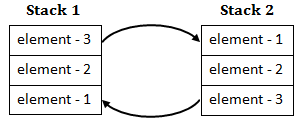Step-2: Once the complete stack-1 gets copied to Stack-2, then we can simply call pop() on s2, it will remove the element-1.
So, A queue can be implemented using 2 stacks.
 Question 15

Considering 0-address instructions machine, what will be the top of the stack after executing the following sequence of instructions?

PUSH 15, PUSH 4, PUSH 6, MULT, PUSH 30, ADD, ADD

 A 30 B 69 C 54 D 10
Data-Structures       Queues-and-Stacks       JT(IT) 2018 PART-B Computer Science
Question 15 Explanation:
Initially stack is empty. We are using last in first out strategy.
Step-1: PUSH 15,PUSH 4 and PUSH 6 from bottom to top. Now top of the stack value is 6.
Step-2: Perform MULT operation. 6*4=24. Now present stack values are from bottom is 15 and 24.
Step-3: Next again PUSH 30. Now top of the stack is 30.
Step-4: Perform ADD operation. 30+24=54
Step-5: Now present stack values are from bottom is 15 and 54. Perform ADD operation.
Step-6: 15+54=69
 Question 16
If queue is implemented using arrays, what would be the worst run time complexity of queue and dequeue operations?
 A O(n), O(n) B O(n), O(1) C O(1), O(n) D O(1), O(1)
Data-Structures       Queues-and-Stacks       Nielit Scientific Assistance IT 15-10-2017
Question 16 Explanation:
Everyone thought that answer in worst case time is O(n) for both the cases but using circular queue, we can implement in constant amount of time.
 Question 17
Which of the following applications may use a stack?
(a) Parenthesis balancing program
(b) Process scheduling operating system
(c) Conversion of infix arithmetic expression to postfix form
 A (a) and (b) B (b) and (c) C (a) and (c) D (a),(b) and (c)
Data-Structures       Queues-and-Stacks       KVS 22-12-2018 Part-B
Question 17 Explanation:
Process scheduling operating system is application of queue.
 Question 18
Given the list of tasks in Col-A and list of data structure in Col-B. Identify the best matchA (i) (iii) (ii) B (ii) (i) (iii) C (iii) (ii) (i) D (ii) (iii) (i)
Data-Structures       Queues-and-Stacks       KVS 22-12-2018 Part-B
Question 18 Explanation:
→ Recursion is implemented stack
→ Scheduling can be implemented using Queue.
→ The inorder traversal of binary search gives sorting order.
 Question 19
For which of the following tasks, stack is not suitable data structure?
(a) Binary search in an array
(b) Breadth first search
(c) Implementing function calls
(d) Process scheduling
 A (b) and (d) B (b) and (c) C (a) and (c) D (c) and (d)
Data-Structures       Queues-and-Stacks       KVS 22-12-2018 Part-B
Question 19 Explanation:
Breadth first search and Process scheduling can be implemented by using Queue.
Binary search can be implemented by using recursion. So Stack is suitable for implementing function calls.
 Question 20
When a circular queue is implemented in an array of the following condition holds when there is only one element in the queue?
 A Front=rear=null B Front=Rear!=null C Front=Rear+1 D Front=Rear-1
Data-Structures       Queues-and-Stacks       KVS 22-12-2018 Part-B
Question 20 Explanation:
Front and rear initially points to same location and once rear is incremented by 1 it means element is added and front is used to delete so here we don't need front for 1 element in the queue.
 Question 21
Which of the following data structures is most suitable for evaluating postfix expressions?
 A Tree B Stack C Linked List D Queue
Data-Structures       Queues-and-Stacks       KVS DEC-2017
Question 21 Explanation:
Postfix Expression are usually converted from infix Expression using Stack Data structure.
Algorithm
We have Postfix[ ] Array, Stack, Infix[ ] Array
1. Scan the given expression (Infix ) from Left to Right [ one character at a time].
2. Check Whether the given character is an operator [+, -, *, /, ^ etc] or operand.
3. If it is an operand, then copy it in the Prefix Array.
4. If it is a operator then,
1. Check whether, Stack is empty or not
2. If it is empty then, push the operator in the stack and go to step
3. If Stack is not empty then compare the precedence of Top of stack with operator.
4. If the Top of Stack has higher or equal precedence then pop it and copy in the postfix array.
5. If the operator has higher precedence, push it in the stack and go to step 5.
6. If Stack is not empty go back to step 4(1).
5. Continue solving the expression in usual manner until the expression come to end.
6. Pop the remaining operand in the stack and copy it to postfix array.
 Question 22
Remove procedure is implemented using
 A String B Queue C Stack D Linked list
Data-Structures       Queues-and-Stacks       KVS DEC-2017
Question 22 Explanation:
→ Every processor provides us with some form of call instruction, which pushes the address of the next instruction on the stack and transfers control to the address specified by the call.
→ When the called procedure is done, it issues a return instruction, which pops the address from the top of the stack and transfers control there.
→ That’s just the basic processor mechanism that makes it easy to implement procedure calls. The actual details of identifying the parameters, placing them on the stack, executing a call instruction are up to the compiler.
→ In the called function, the compiler is responsible for ensuring any registers that may be clobbered are saved, allocating stack space for local variables, and then restoring the registers and stack prior to a return.
 Question 23
The five items P<Q<R
 A S B P C Q D R
Data-Structures       Queues-and-Stacks       KVS DEC-2017
Question 23 Explanation:
Step-1: Initially P,Q,R,S and T are pushed in a stack. The stack elements will be placed in LIFO manner. It beans that bottom of the stack value from P to T.
Step-2: Then we have to pop 4 elements. So, present having only one element,that is P.
Step-3: 4 elements we have to insert into a Queue. Queue follows FIFO manner.
Step-4: Again we have to delete two elements from queue and inserted into stack.
Step-5: The inserted elements are T and S.
Step-6: Final pop element is S.
Step-7: Stack having only two elements. P and T.
 Question 24
Consider a single dimension array A[n], which houses two stacks as shown in the figures. A is the bottom of stack A and A[n] is the bottom of stack B.If nA is the number of elements in stack A and nB is the number of elements in stack B, then overflow occurs when Push Operation is performed on either stack and
 A nA=nB B nA=nB=n C nA+nB=n D nA+nB>=n
Data-Structures       Queues-and-Stacks       KVS 30-12-2018 Part B
Question 24 Explanation:
→nA is the number of elements in stack A and nB is the number of elements in stack B, Where “n” is total elements we can insert into stack.
→The sum of the nA and nB is equal to the number of elements there is no possibility of inserting element into stack.
 Question 25
Translation of infix string a+b*c-d/e*h to postfix form is
 A abc*+deh/*- B ab+c*de/h*- C (a b c*)+(de/h*-) D abc*+de/h*-
Data-Structures       Queues-and-Stacks       KVS 30-12-2018 Part B
Question 25 Explanation:
The infix string a+b*c-d/e*h.
=a+[bc*]-d/e*h // Select highest priority operators and convert into postfix form,If same priority then go for associativity
=a+[bc*]-[de/]*h
=a+[bc*]-[de/h*]
=[abc*+]-[de/h*]
=abc*+de/h*-
 Question 26
Queue is an appropriate data structures for
a) Implementation breadth first search
b) Implementation depth first search
c) Process scheduling
Which of the following is the correct option?
 A a and b B a and c C b and c D a,b and c
Data-Structures       Queues-and-Stacks       KVS 30-12-2018 Part B
Question 26 Explanation:
→Various Queues like Ready queue,Job Queue , Waiting Queue and so on are used in the operating system for process scheduling.
→Queue is also used for Breadth first traversal also
 Question 27
The seven elements A, B, C, D, E, F and G are pushed onto a stack in reverse order, i.e., starting from G. The stack is popped five times and each element is inserted into a queue.Two elements are deleted from the queue and pushed back onto the stack. Now, one element is popped from the stack. The popped item is ________.
 A A B B C F D G
Data-Structures       Queues-and-Stacks       UGC NET CS 2017 Jan -paper-2
Question 27 Explanation:
Step-1: Insert all elements into stack in reverse order then the stack is look likeStep-2: Stack is pooped five elements then stack is look like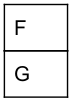Step-3: Popped five elements are inserted into a queue then queue is look likeStep-4: Two elements are deleted from the queueStep-5: deleted queue elements are pushed back onto the stack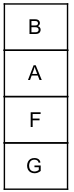Top of the stack is B.
 Question 28
Consider the following statements:
S1: A queue can be implemented using two stacks.
S2: A stack can be implemented using two queues.
Which of the following is correct?
 A S ​ 1​ is correct and S ​ 2​ is not correct. B S 1​ ​ is not correct and S 2​ ​ is correct. C Both S​ 1 and S​ 2​ are correct. D Both S​ 1​ and S​ 2​ are incorrect.
Data-Structures       Queues-and-Stacks       UGC NET CS 2016 Aug- paper-2
Question 28 Explanation:
→ Implementing queue by using two stack:
(1) When calling the enqueue method, simply push the elements into the stack 1.
(2) If the dequeue method is called, push all the elements from stack 1 into stack 2, which reverses the order of the elements. Now pop from stack 2.
→ A stack can be implemented using two queues
 Question 29
Consider the following operations performed on a stack of size 5 : Push (a); Pop() ; Push(b); Push(c); Pop(); Push(d); Pop();Pop(); Push (e) Which of the following statements is correct?
 A Underflow occurs B Stack operations are performed smoothly C Overflow occurs D None of the above
Data-Structures       Queues-and-Stacks       UGC NET CS 2016 July- paper-2
Question 29 Explanation:
Here, stack size is 5. Stack operations are explained in step by step.Question 30
Which of the following is not an inherent application of stack?
 A Implementation of recursion B Evaluation of a postfix expression C Job scheduling D Reverse a string
Data-Structures       Queues-and-Stacks       UGC NET CS 2016 July- paper-2
Question 30 Explanation:
Applications
1. Implementation of recursion
2. Evaluation of a postfix expression
3. Expression evaluation and syntax parsing
4. Backtracking
5. Compile time memory management
6. Reverse a string
 Question 31
In how many ways can the string A ∩ B – A ∩ B – A be fully parenthesized to yield an infix expression?
 A 15 B 14 C 13 D 12
Data-Structures       Queues-and-Stacks       UGC NET CS 2016 July- paper-2
Question 31 Explanation:
In this problem look very difficult to solve, but problem is following standard formula only.
Step-1: Total number of letters/operands with duplication is 5.
Step-3: To find total number of ways, we have catalan formula.
Here, n=5
Catalan number = (2n)! / (n! * (n+1)!)
= (2*5)! / (5! * (5+1)!)
= 10! / (5! * 6!)
= 14
Note: We used catalan formula because they given in fully parenthesized to yield an infix expression.
 Question 32
The initial configuration of queue is a, b, c, d. ‘​ a’​ is at the front. To get the configuration d, c, b, a how many deletions and additions required :
 A 2 deletions, 3 additions B 3 deletions, 2 additions C 3 deletions, 4 additions D 3 deletions, 3 additions
Data-Structures       Queues-and-Stacks       UGC NET CS 2005 Dec-Paper-2
Question 32 Explanation:
Initially the queue order isStep-1: Delete ‘a’ from queue. The deletion operation performs First In First Out.We will perform the deletion at front end
After deleting queue will becomeStep-3: Delete ‘b’ from queue. The deletion operation performs First In First Out.
After deleting queue will become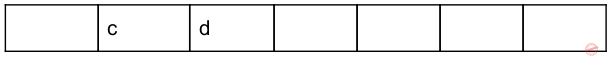Step-3: Delete ‘c’ from queue. The deletion operation performs First In First Out.
After deleting queue will becomeStep-4: Insert the element “c” into the queue, We will perform the insertion at rear end
The elements of queue after inserting the element ”c” areStep-5: Insert the element “b” into the queue,then the elements of queue after inserting the element ”b” arestep-6: Insert the element “a” into the queue,then the elements of queue after inserting the element ”a” are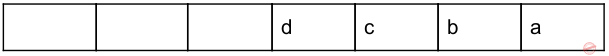→ Step-1,step-2 and step-3 are deletion operations and step-4,step-5 and step-6 are deletions operations.
→ So total 3 deletions and 3 insertions are required to get required configuration.
 Question 33
What is the time required to insert an element in a stack with linked implementation ?
 A O (log​ 2​ n) B O (n) C O (n log​ 2​ n) D O (1)
Data-Structures       Queues-and-Stacks       UGC NET CS 2005 june-paper-2
Question 33 Explanation:Question 34
If the postfix form of a string is ABC+ - D*, the actual string is :
 A (A-(B+C))*D B ((A-B)+C)*D C ((A+B)-C)*D D (A+(B-C))*D
Data-Structures       Queues-and-Stacks       UGC NET CS 2005 june-paper-2
Question 34 Explanation: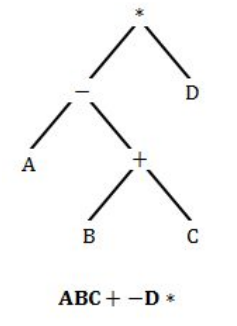Question 35
What is the time required to insert an element in a stack with linked implementation ?
 A O (log​ 2​ n) B O (n) C O (n log​ 2​ n) D O (1)
Data-Structures       Queues-and-Stacks       UGC NET CS 2006 Dec-paper-2
Question 35 Explanation:
Average case in linked list: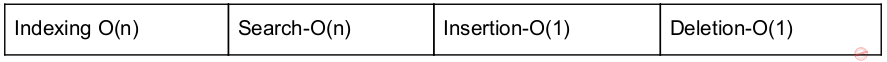Worst case in linked list:Question 36
The equivalent postfix expression for d/(e +f) +b*c
 A defbc/++* B def+/bc+* C def+/bc *+ D None of these
Data-Structures       Queues-and-Stacks       UGC NET CS 2006 Dec-paper-2
Question 36 Explanation: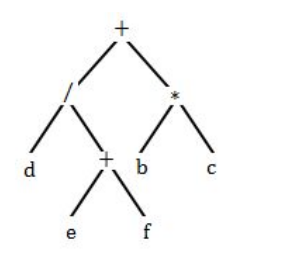Postfix expression: d ef + / bc * +
 Question 37
If the post-fix form of a string is ABC+ -D*, The actual string is :
 A (A-(B+C))*D B ((A-B)+C)*D C ((A+B)-C)*D D (A+(B-C)*D)
Data-Structures       Queues-and-Stacks       UGC NET CS 2006 June-Paper-2
Question 37 Explanation:Question 38
Application of data structure queue is :
 A Level wise printing of tree B Implementation of priority queues C Function call implementation D Depth first search in a graph
Data-Structures       Queues-and-Stacks       UGC NET CS 2006 June-Paper-2
Question 38 Explanation:
Application of data structure queue is implementation of priority queues. In a priority queue, an element with high priority is served before an element with low priority. In some implementations, if two elements have the same priority, they are served according to the order in which they were enqueued, while in other implementations, ordering of elements with the same priority is undefined. While priority queues are often implemented with heaps.
Applications:
1.Bandwidth management
2.Discrete event simulation
3.Dijkstra's algorithm
4.Huffman coding
5.Best-first search algorithms
6.ROAM triangulation algorithm
7.Prim's algorithm for minimum spanning tree
 Question 39
How many PUSH and POP operations will be needed to evaluate the following expression by reverse polish notation in a stack machine (A * B) + (C * D/E) ?
 A 4 PUSH and 3 POP instructions B 5 PUSH and 4 POP instructions C 6 PUSH and 2 POP instructions D 5 PUSH and 3 POP instructions
Data-Structures       Queues-and-Stacks       UGC NET CS 2014 Dec-Paper-2
Question 39 Explanation:
Given infix notation, we have to change infix notation into postfix notation.
after converting postfix notation the notations are ab* cde /* +Total 5 PUSH and 4 POP operations performed.
 Question 40
Given an empty stack, after performing Push(1), Push(2), Pop, Push(3), Push(4), Pop, Pop, push(5), Pop. what is the value of the top of the stack ?
 A 4 B 3 C 2 D 1
Data-Structures       Queues-and-Stacks       UGC NET CS 2012 Dec-Paper-2
Question 40 Explanation: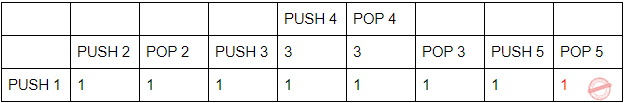Question 41
What is the value of the postfix expression ?
a b c d + – ∗ (where a = 8, b = 4, c = 2 and d = 5)
 A –3/8 B –8/3 C 24 D –24
Data-Structures       Queues-and-Stacks       UGC NET CS 2013 Dec-paper-2
Question 41 Explanation:Question 42
The postfix expression AB + CD – * can be evaluated using a
 A stack B tree C queue D linked list
Data-Structures       Queues-and-Stacks       UGC NET CS 2012 June-Paper2
Question 42 Explanation:Question 43
The value of postfix expression :
8 3 4 + – 3 8 2 / + * 2 \$ 3 + is
 A 17 B 131 C 64 D 52
Data-Structures       Queues-and-Stacks       UGC NET CS 2013 June-paper-2
Question 43 Explanation: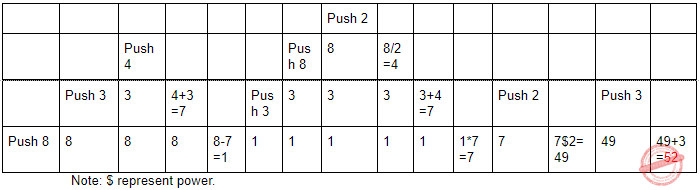Question 44
Consider the following statements for priority queue :
S1 : It is a data structure in which the intrinsic ordering of the elements does determine the result of its basic operations.
S2 : The elements of a priority queue may be complex structures that are ordered on one or several fields.
Which of the following is correct ?
 A Both S1 and S2 are incorrect. B S1 is correct and S2 is incorrect. C S1 is incorrect and S2 is correct. D Both S1 and S2 are correct
Data-Structures       Queues-and-Stacks       UGC NET CS 2013 June-paper-2
Question 44 Explanation:
S1: TRUE: It is a data structure in which the intrinsic ordering of the elements does determine the result of its basic operations.
S2: TRUE: The elements of a priority queue may be complex structures that are ordered on one or several fields.
 Question 45
If we have six stack operations pushing and popping each of A, B and C such that push(A) must occur before push(B) which must occur before push(C), then A, C, B is a possible order for the pop operations,since this could be our sequence : push(A), pop(A), push(B), push(C), pop(C), pop(B). Which one of the following orders could not be the order the pop operations are run, if we are to satisfy the requirements described above ?
 A ABC B CBA C BAC D CAB
Data-Structures       Queues-and-Stacks       UGC NET CS 2010 June-Paper-2
Question 45 Explanation:Option-A: According to constraint,
Push(A) then Pop(A)
Push(B) then Pop(B)
Push(C) then Pop(C)
Then the possible pop sequence is ABC. So, it is TRUE
Option-B: According to given constraint, Push(A),Push(B) and Push(C) then Pop(C),
Pop(B) and Pop(A). The possible Pop sequence is CBA. So, it is TRUE
Option-C: According to given constraint, Push(A) and Push(B) then Pop(B) and Pop(A)
then push(C) and Pop(C). The possible Pop sequence is BAC. So, it is TRUE
Option-D: This is not possible.
 Question 46
What is the most appropriate data structure to implement a priority queue ?
 A Heap B Circular array C Linked list D Binary tree
Data-Structures       Queues-and-Stacks       UGC NET CS 2010 June-Paper-2
Question 46 Explanation:
→ Priority queue is an abstract data type which is like a regular queue or stack data structure, but where additionally each element has a "priority" associated with it.
→ In a priority queue, an element with high priority is served before an element with low priority.
→ In some implementations, if two elements have the same priority, they are served according to the order in which they were enqueued, while in other implementations, ordering of elements with the same priority is undefined.
→ While priority queues are often implemented with heaps, they are conceptually distinct from heaps. A priority queue is an abstract concept like "a list" or "a map".
→ just as a list can be implemented with a linked list or an array, a priority queue can be implemented with a heap or a variety of other methods such as an unordered array.
 Question 47
Queue is a __________ list.
 A LIFO B LILO C FILO D FIFO
Data-Structures       Queues-and-Stacks       UGC NET CS 2009-June-Paper-2
Question 47 Explanation:
Queue is FIFO(First In First Out) and Stack is LIFO(Last In First Out).
 Question 48
At a hill station, the parking lot is one long driveway snaking up a hill side. Cars drive in and park right behind the car in front of them, one behind another. A car can’t leave until all the cars in front of it have left. Is the parking lot more like
 A An array B A stack C A queue D A linked list
Data-Structures       Queues-and-Stacks       UGC NET CS 2009 Dec-Paper-2
Question 48 Explanation:
The above data clearly using first in first out(FIFO) condition. FIFO we are using queue data structure.
 Question 49
If (rear == maxsize -1) rear=0; else rear=rear+1; is required in :
 A Circular Queue B Linear Queue C Stack D Deque
Data-Structures       Queues-and-Stacks       UGC NET CS 2008 Dec-Paper-2
 Question 50
Which of the following data structures is most efficient in terms of both space and time to reverse a string of characters ?
 A Linked list B Stack C Array D Tree
Data-Structures       Queues-and-Stacks       UGC NET CS 2008-june-Paper-2
Question 50 Explanation:
To print values in reverse order we are preferring stack data structure only. Stack using Last in first out(LIFO) procedure.Question 51
Which of the following data structure is used to implement recursion ?
 A Arrays B Stacks C Queues D Linked lists
Data-Structures       Queues-and-Stacks       UGC NET CS 2007-Dec-Paper-2
Question 51 Explanation:
→ Stack is used to implement recursion. It uses Last in first out procedure.
→ Recursion is one the application of stack. It supports many applications.
Applications:
1. Expression Evaluation
2. Expression Conversion
3. Syntax Parsing
4. Backtracking
5. Parenthesis Checking
6. String Reversal
 Question 52
The equivalent postfix express for d/(e+f) + b*c is :
 A defbc/+ + B def+/bc+* C def+/bc*+ D None of these
Data-Structures       Queues-and-Stacks       UGC NET CS 2007 June-Paper-2
Question 52 Explanation:Question 53
Application of data structure is queue is :
 A Level wise printing of tree. B Implementation of priority queues. C Function call implementation D Depth first search in a graph
Data-Structures       Queues-and-Stacks       UGC NET CS 2007 June-Paper-2
Question 53 Explanation:
Queue applications:
1. CPU scheduling
2. Disk Scheduling
3. synchronization(IO Buffers, pipes, file IO, etc.)
4. Breadth First search
5. Implementation of priority queues.
There are 53 questions to complete.
PHP Code Snippets Powered By : XYZScripts.com